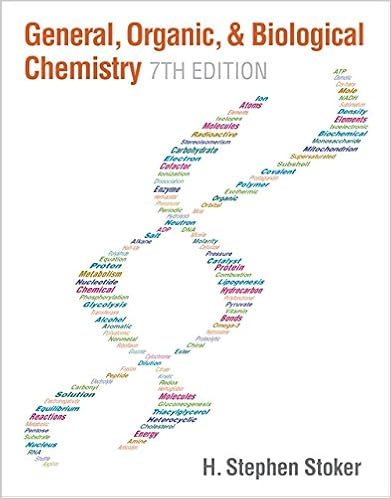The molecule acetone is CH 3 COCH 3 If a sample contains...

• Test Prep
• 4

This preview shows page 1 - 2 out of 4 pages.

We have textbook solutions for you!
The document you are viewing contains questions related to this textbook.The document you are viewing contains questions related to this textbook.
Chapter 15 / Exercise 2
General, Organic, & Biological Chemistry
StokerExpert Verified
1070 Week 1-to-2 check-in quiz 1) The molecule acetone is CH 3 COCH 3 . If a sample contains half a mole of acetone molecules, which of the following statements is true: a) The sample contains 1.0 mole of C atoms and 3.0 moles of H atoms. b) The sample contains 1.5 moles of C atoms and 3.0 moles of H atoms. CORRECT ANSWER c) The sample contains 1.0 mole of C atoms and 6.0 moles of H atoms. d) The sample contains 1.5 moles of C atoms and 6.0 moles of H atoms.
2) The molecule aspirin has the chemical formula C 9 H 8 O 4 . If a sample contains two moles of aspirin, which of the following statements is true: CORRECT
ANSWER d) The sample contains 1/18 moles of C atoms and 1/16 moles of H atoms. 3) A new molecule is composed of S and a new element Z with molecular formula SZ 4 . There are two samples of this new molecule. In the first sample there are 0.6 g of S atoms and 1.0 g of Z atoms. In the second sample there are 3.0 g of Z atoms. Which of the following is true?
We have textbook solutions for you!
The document you are viewing contains questions related to this textbook.The document you are viewing contains questions related to this textbook.
Chapter 15 / Exercise 2
General, Organic, & Biological Chemistry
StokerExpert Verified
•••